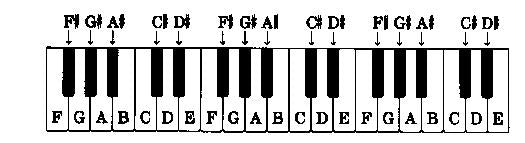# Chapter 2 Piano Keys Scales

Piano Theory I Notes

The Natural Music Notes : C   D     E    F     G   A    B   C        These are Full Notes/ Tones

SA RE GA MA PA DA NI SA

Complete Chromatic Sequence of Notes:  C C# D D# E F F# G G# A A# B CPiano Scales formation & Key Pad

Scale is a set of Notes in a particular order.

Types of Scales:

• Major Scales
• Minor Scales
• Pentatonic Scales
• Harmonic Scales
• Diminished Scales etc.

Major Scale formation:

Major Scale = R  w w h w w w h

R = Root Note =  the main note of the scale

w = whole step = 2 notes distance ,

h = half step = immediate next note

For example,

R        w        w     h       w      w      w     h

C Major scale =   C       D         E     F       G      A        B    C

w = D = is 2 notes away from C i.e. C C# and then D

similarly, h = F = next note from E so half step.

Minor Scale formation:

Minor Scale = R  w h w w h w w

R = Root Note =  the main note of the scale

w = whole step = 2 notes distance ,

h = half step = immediate next note

For example,

R        w        h       w      w     h      w     w

C Minor scale =   C       D        D#     F       G     G#    A#    C

In the same way using respective formulas one can find the notes in the other scales. In the next chapter we will see the Major Minor scale notes.

"Join the most economical and most productive Casio / Keyboard / Piano /Synth classes in Pune."